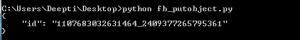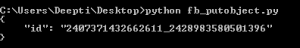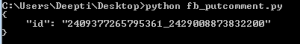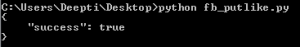Related Articles
• Difficulty Level : Basic
• Last Updated : 26 Jul, 2019

Prerequisites: Facebook API Set-1, Set-2, Set-3

1. put_object
2. put_like
3. put_comment
1. put_object: Writes the mentioned object to the Facebook Social Graph connected to the given parent.
Parameters:
1. parent_object: A string specifying the parent of the new object. For example- Post is the parent of comment, when we want to add a new comment or when we want to add a new photo, User profile is the parent of the photo.
2. connection name: A string specifying the connection or edges between the objects.

Example#1: Post a message with a link to the active user wall.

 `import` `json``import` `facebook`` ` `def` `main():``    ``token ``=` `"Please replace this with "``me" ``or` `your Access Token(``for` `Posting on your wall)\``             ``or` `with PAGE Access Token(``for` `posting on Page)"`` ` `    ``graph ``=` `facebook.GraphAPI(token)``    ``message ``=` `graph.put_object(parent_object ``=``"me"``, ``              ``connection_name ``=``"feed"``,``              ``message ``=``"Hello this is a great website for various Computer Science Topics."``,``              ``link ``=``"https://www.geeksforgeeks.com"``)`` ` `    ``print``(json.dumps(message, indent ``=` `4``))`` ` `if` `__name__ ``=``=` `'__main__'``:``    ``main()``import` `json``import` `facebook`` ` `def` `main():``    ``token ``=` `"Please replace this with your PAGE ACCESS TOKEN"``    ``graph ``=` `facebook.GraphAPI(token)``commenttopost ``=` `graph.put_object(parent_object ``=``"PAGEID_POSTID"``,``                ``connection_name ``=``"comments"``,``                ``message ``=``"Please share and Like the website for content on Computer Science"``)``    ``print``(json.dumps(commenttopost, indent ``=` `4``))`` ` `if` `__name__ ``=``=` `'__main__'``:``    ``main()`2. put_comment: This methods facilitates to write a message as comment to an object.
Parameters:
1. object_id: A string unique for a particular resource.
2. message: A string to be posted as comment.
 `import` `json``import` `facebook`` ` `def` `main():``    ``token ``=` `"Please replace this with your PAGE ACCESS TOKEN"``    ``graph ``=` `facebook.GraphAPI(token)``    ``putcomment ``=` `graph.put_comment(object_id ``=``"PAGEID_POSTID"``,``                 ``message ``=``"This is a very good website for Computer Science Topics"``)`` ` `    ``print``(json.dumps(putcomment, indent ``=` `4``))`` ` `if` `__name__ ``=``=` `'__main__'``:``    ``main()`3. put_like: Add like to a given object
Parameters:
object_id: A string that is a unique id for the particular resource.
 `import` `json``import` `facebook`` ` `def` `main():``    ``token ``=` `"Please replace this with your PAGE ACCESS TOKEN"``    ``graph ``=` `facebook.GraphAPI(token)``    ``putlike ``=` `graph.put_like(object_id ``=``"PAGEID_POSTID"``)``    ``print``(json.dumps(putlike, indent ``=` `4``))`` ` `if` `__name__ ``=``=` `'__main__'``:``    ``main()`My Personal Notes arrow_drop_up